Math Calculators, Lessons and Formulas

It is time to solve your math problem

mathportal.org
• Geometry
• Rectangle

# Rectangle

ans:
syntax error
C
DEL
ANS
±
(
)
÷
×
7
8
9
4
5
6
+
1
2
3
=
0
.
auto next question
calculator
•  Question 1: 1 pts Find the area of the rectangle shown on the picture.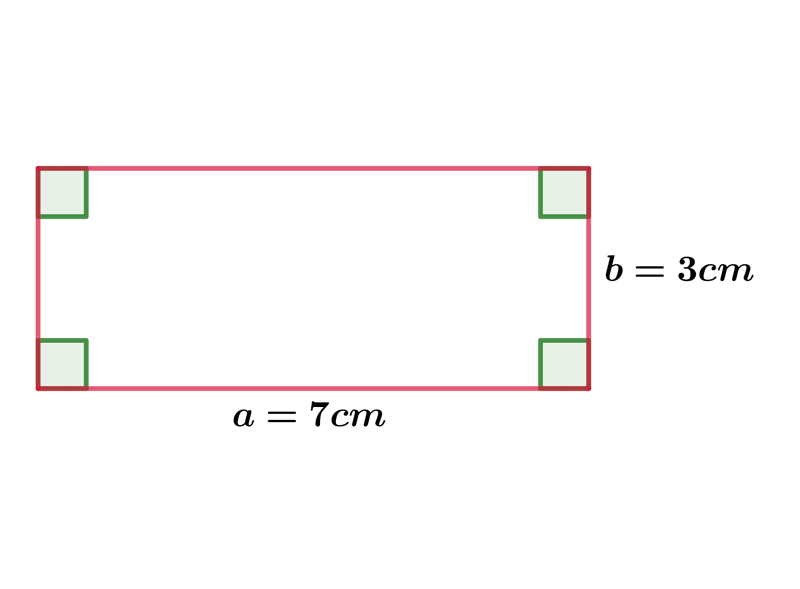$A=$
•  Question 2: 1 pts Find the length of the rectangle's diagonal.$d=60cm$ $d=17cm$ $d=7cm$ $d=13cm$
•  Question 3: 1 pts The perimeter of the rectangle shown on the picture is $6cm.$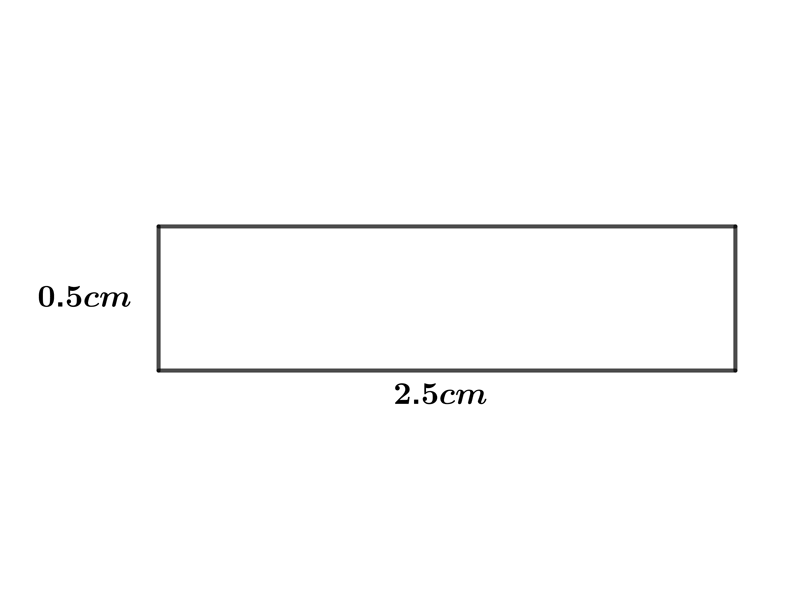•  Question 4: 1 pts Find the length of the diagonal of a rectangle whose sides are $7 cm$ and $24 cm.$
 $d=25cm$ $d=31cm$ $d=17cm$ $d=22cm$
•  Question 5: 2 pts Find the length of the diagonal of a rectangle whose one side is $a=12 cm$, and the area of rectangle is $A=108cm^{2}$.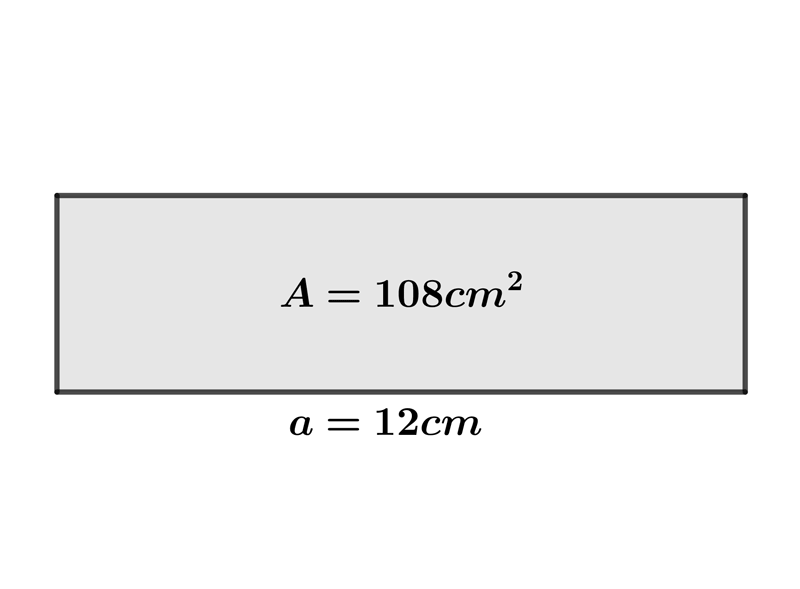$d=$
•  Question 6: 2 pts Find the perimeter of the rectangle whose length is $8 cm$ and a diagonal $4\sqrt{5} cm.$
 $P=14cm$ $P=24cm$ $P=18cm$ $P=21cm$
•  Question 7: 2 pts Find the area of the rectangle shown on the picture.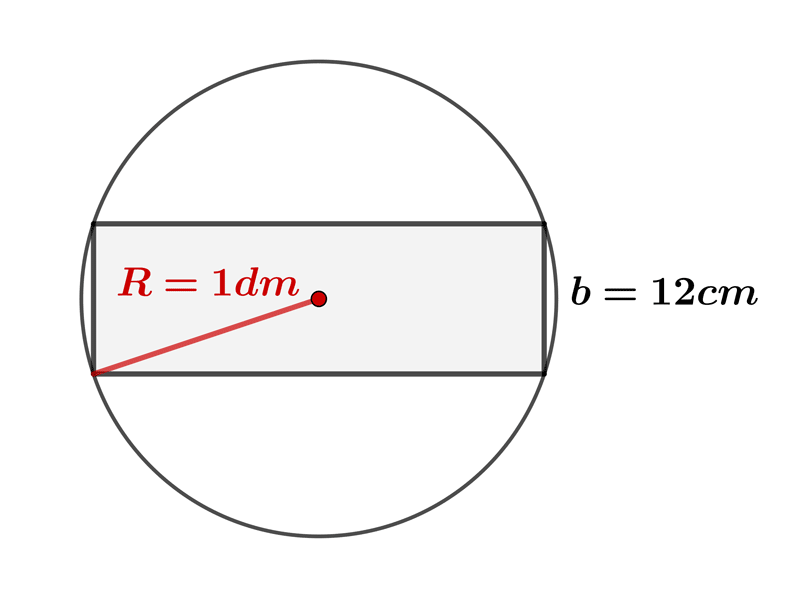$A=$
•  Question 8: 2 pts A rectangle has one side double in length than the other side. If the rectangle's diagonal is $10\sqrt{5}cm$, what would be the area of the rectangle?
 $A=144cm^{2}$ $A=196cm^{2}$ $A=200cm^{2}$ $A=169cm^{2}$
•  Question 9: 3 pts Rectangle $ABCD$ is shown on the picture. Find the distance between point $B$ and diagonal $AC.$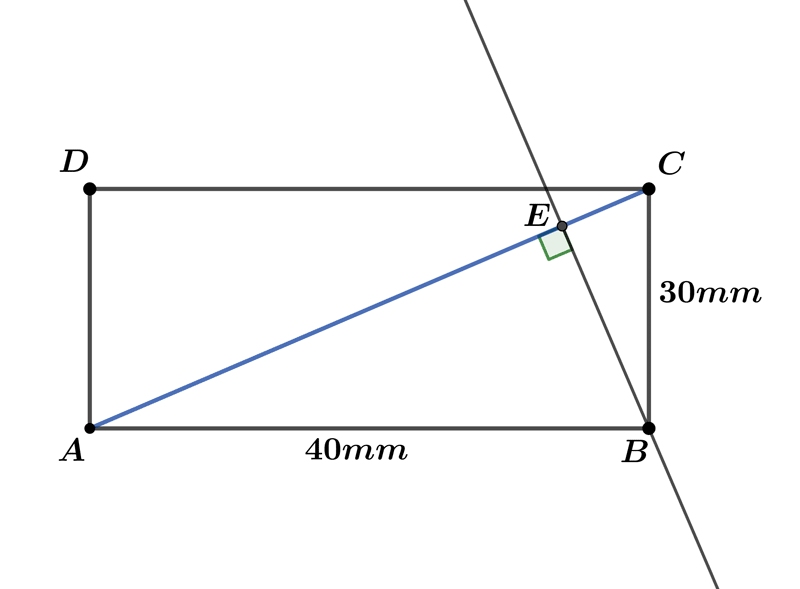$BE=$
•  Question 10: 3 pts The perimeter of the quadrilateral $MNPQ$ who is inscribed in the rectangle $ABCD$ is $8\sqrt{5}.$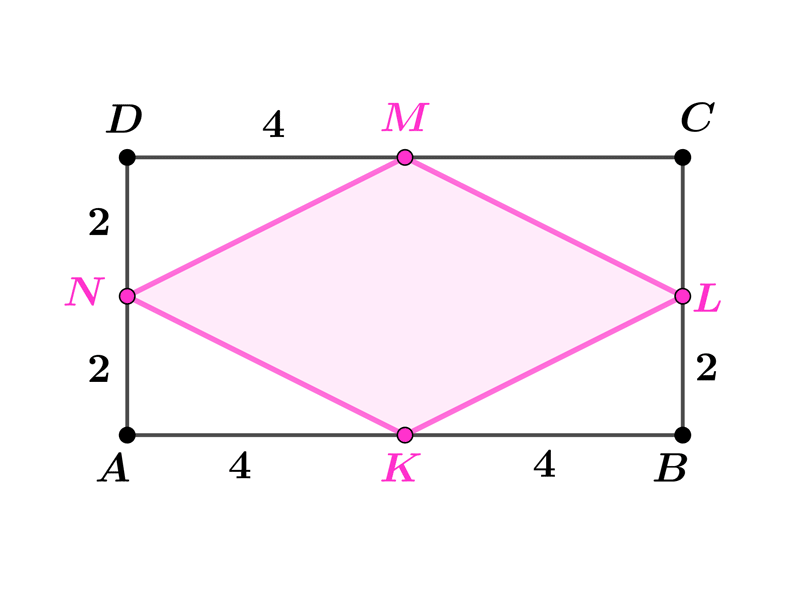•  Question 11: 3 pts The area of the quadrilateral $MNPQ$ who is inscribed in the rectangle $ABCD$ is $8.$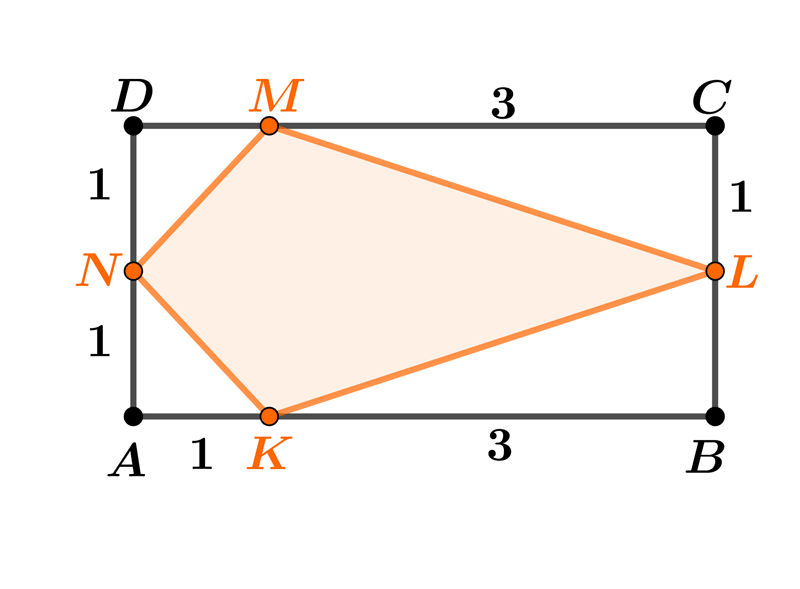•  Question 12: 3 pts Find the area of the shape shown on the picture.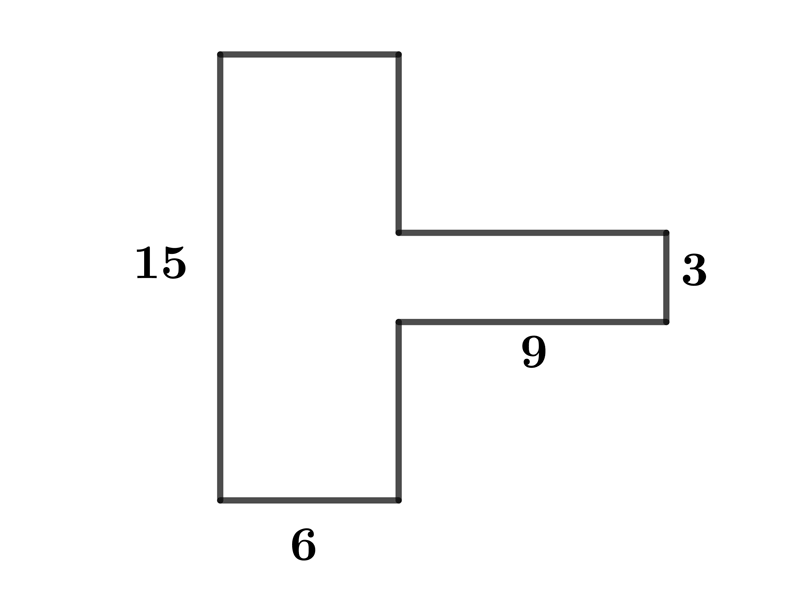$A=108$ $A=96$ $A=118$ $A=117$